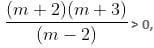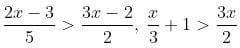CAT  >  Practice Questions Level 2: Inequalities - 2

# Practice Questions Level 2: Inequalities - 2 - Notes | Study Level-wise Practice Questions for CAT Preparation - CAT

 1 Crore+ students have signed up on EduRev. Have you?

This EduRev document offers 10 Multiple Choice Questions (MCQs) from the topic Inequalities (Level - 2). These questions are of Level - 2 difficulty and will assist you in the preparation of CAT & other MBA exams. You can practice/attempt these CAT Multiple Choice Questions (MCQs) and check the explanations for a better understanding of the topic.

Question for Practice Questions Level 2: Inequalities - 2
Try yourself:How many pairs of integers satisfy the inequality |x| + |y| = 7?

Question for Practice Questions Level 2: Inequalities - 2
Try yourself:It is given that m and n are two real numbers. Which of the following is true for all possible values of m and n?

Question for Practice Questions Level 2: Inequalities - 2
Try yourself:What is the sum of all possible values of m in the given inequality?m is an integer, it is known that m ≤ 99.

Question for Practice Questions Level 2: Inequalities - 2
Try yourself:The number of solutions of the equation 2x + y = 40, where both x and y are positive integers and x ≤ y, is

Question for Practice Questions Level 2: Inequalities - 2
Try yourself:If 2y - x > 2x - y, and 0 ≤ y ≤ 20, how many non-negative integer pairs of x and y satisfy the given inequalities?

Question for Practice Questions Level 2: Inequalities - 2
Try yourself:Consider the following system of inequalities:Which of the following options best describes the value of x?

Question for Practice Questions Level 2: Inequalities - 2
Try yourself:What is the least possible value of c - a if a < b < c and b - a > 5, where a is an even integer and b and c are odd integers?

Question for Practice Questions Level 2: Inequalities - 2
Try yourself:How many pairs of consecutive odd positive integers, smaller than 18 and having their sum as more than 20, are possible?

Question for Practice Questions Level 2: Inequalities - 2
Try yourself:If (1 + 3c), 1 and (1 - c) are the three sides of a triangle, then

Question for Practice Questions Level 2: Inequalities - 2
Try yourself:If x satisfies the inequality |x − 1| + |x − 2| + |x − 3| ≥ 6, then:

The document Practice Questions Level 2: Inequalities - 2 - Notes | Study Level-wise Practice Questions for CAT Preparation - CAT is a part of the CAT Course Level-wise Practice Questions for CAT Preparation.
All you need of CAT at this link: CAT

## Level-wise Practice Questions for CAT Preparation

277 docs
 Use Code STAYHOME200 and get INR 200 additional OFF

## Level-wise Practice Questions for CAT Preparation

277 docs

### How to Prepare for CAT

Read our guide to prepare for CAT which is created by Toppers & the best Teachers

Track your progress, build streaks, highlight & save important lessons and more!

,

,

,

,

,

,

,

,

,

,

,

,

,

,

,

,

,

,

,

,

,

;